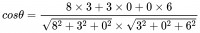• A: `['编程', '摄影']`
• B: `['编程', '旅游']`

``['编程', '摄影', '旅游']``

• A: `[8, 3, 0]`
• B: `[3, 0, 6]```````<?php

\$a = [8, 3, 0];
\$b = [3, 0, 6];

\$molecular = 0;
\$aSqr = 0;
\$bSqr = 0;
foreach (\$a as \$i => \$v) {
\$molecular += \$v * \$b[\$i];
\$aSqr += pow(\$v, 2);
\$bSqr += pow(\$b[\$i], 2);
}

var_dump(\$molecular/(sqrt(\$aSqr)*sqrt(\$bSqr)));``````